# Hypercohomology

<]]>

<]]>
• ## Online References

For hypercohomology of complexes not bounded from below, see Voevodsky and Suslin: Bloch-Kato conj and motivic cohomology with finite coeffs, file in Voevodsky folder, pp 4.

For hypercohomology of pointed simplicial sheaves, see Appendix 1 of Voevodsky: Motivic cohomology with Z/2 coeffs, published version.

<]]>
• ## Paper References

Lubkin has a section on relative hypercohomology.

<]]>
• ## Definition

Ref: Discussion with Scholl, Oct 2007.

To compute ordinary Sheaf cohomology of a sheaf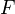on some space/scheme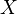(in general a site withas the final object, I guess), one takes an injective resolution of the sheaf,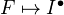, applies the global section functor to get a complex of abelian groups, and then takes the cohomology of that complex. One shows that a homotopy equivalence between injective resolutions gives same cohomology.

Let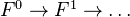be a complex of sheaves. Then we can find a quasi-isomorphism of complexes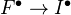to a complex of injectives. (And also, I think, for two such quasi-IMs, there is a homotopy equivalence between the two complexes of injectives, making the whole thing commute.

Now the hypercohomology of the complex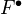is defined by taking the cohomology of the global sections complex of this complex of injectives. Note that we get back usual sheaf cohomology as a special case: And injective resolution is nothing but a quasi-isomorphism from the complex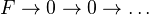.

Memo ("apply blindly"):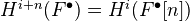("anyone who uses another convention should be shot"). Similarly,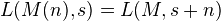.

For any complex of abelian groups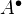, the cohomology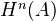is the homotopy classes of maps from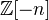to.

<]]>

<]]>

<]]>

<]]>

<]]>

<]]>

<]]>
• ## Some Research Articles

Verdier: Topologie sur les espaces de cohomologie d’un complexe de faisceaux analytiques a cohomologie coherente. Computes hypercohomology (also with compact supports) by constructing a complex of Frechet nuclear spaces with the right topology. This is done in a way that is functorial in bothand.

<]]>

<]]>